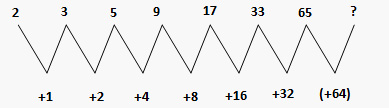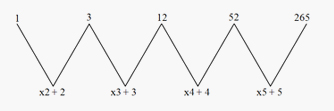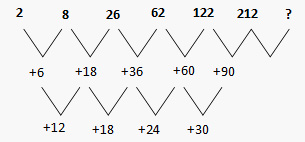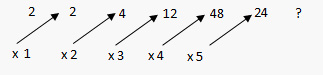# Series Number Sequence: Solved Examples

Directions for questions 1 to 10: Find the missing term in each of the following questions of number series.
Example 1: Identify the next term in the following series 2, 3, 5, 9, 17, 33, 65, ?
Sol: This is one of the simpler kinds of series, as it can be seen that the difference between the numbers goes like this.You can find the right answer in number series by taking the difference between consecutive pairs of numbers, which form a logical series. In this example, the differences between succeeding pairs of numbers are 1,2, 4, 8, 16, and 32. So, the next difference must be 64. 64, if added to the last number 65, gives 129, which is the correct answer.
Example 2: Find the next number in the following series 1, 3, 12, 52, 265, ?.
Sol:The above series involves two operations: multiplication and addition of a number by the same number. The series runs like this: 1 x 2 + 2 = 3, 3 x 3 + 3 = 12, 12 x 4 + 4 = 52, 52 x 5 + 5 = 265. The next number, following this logic should be 265 x 6 + 6 = 1596. In this case, the idea of differences will not help us.
Example 3: Identify the next number in the following series 2, 8, 26, 62, 122, 212,?
Sol: First of all you should try to take the differences. If the first difference does not give anything significant, you can go for the second difference i.e. the difference of the differences.The first differences obviously do not contain any logic. But taking the differences among differences in the first layer as shown above, we see that the logic becomes very clear to us. Following the very same logic, the last addition to last number i.e. 30, should be 6, which makes it 36. Moving backwards, we get 36 + 90 + 212 = 338, which is the correct answer to this particular question.
Example 4: Find the next number 13, 17, 19, 23, 29,?
Sol: This is a series of prime numbers, it can be clearly seen that the numbers given are successive prime numbers. Next prime number would be 31 and that would be the answer.
Example 5: What should come in place of question mark in the following series: 3, 5, 9, 15, 23, 33,?, 59.
Sol: Here, the additive factor is increased by two every time i.e. firstly two is added, then four is added, then six is added and so on. It can be seen that before the question mark, ten has been added, so the next addition would be twelve. Thus, the number would be 33 + 12 = 45.
Example 6: What should come in place of question mark in the following series; 16, 22, 28, 34,?, 46
Sol: In this case, six has been added in all the numbers, to get the next number. First of all: 16 + 6 = 22, then 22 + 6 = 28 and so on. In order to get the next number after 34, 6 would be added to it. So, the question mark would be replaced by 34 + 6 = 40.
Example 7: What should come in place of question mark in the following series: 2, 3, 6, 11, 18, 27, ?
Sol: In this case consecutive odd numbers have been added in the increasing order. Firstly one, then three, then five and nine. So, in order to obtain the next number, eleven will be added. Therefore, the next number is: 27 + 11 = 38.
Example 8: What should be come in place of x in the following series; 2, 2, 4, 12, 48, 240, ?
Sol: In this question, every number is multiplied by the consecutive natural numbers starting from one. This can be understood with the help of the following diagram.The next number, as is clear from the above illustration, is obtained by multiplying the last number 240 by 6. So, the answer is 240 × 6 = 1440.
Example 9: Find the wrong number in the following series:
16  47  64  72  76  78  79
Sol: In this particular example, if you start from the first term, first of all, the difference is thirty one, then the difference is seventeen, and then the difference is eight. So there is no specific logic. Starting from the last term, you can see that firstly, one is added, then two is added, then four is added, then eight, sixteen and thirty two should have been added. So you have got the right clue. So, 16 + 32 i.e. the first number should be 48 and afterwards the series becomes + 16, + 8, + 4 and so on. Thus 47 is the wrong number in this particular series.
Example 10: Find the wrong number in the below mentioned series:
11 23 46 95 191
Sol: In this particular question, the pattern used is x 2 + 1 only. But in the middle when 23 x 2 + 1 = 47 should have been written, 46 is written instead of that. So, 46 is the wrong number in this particular series.